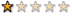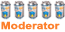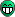## [問題]積分系統

phpBB 2 MOD Support

(發表文章請按照公告格式發表，違者砍文)

ponbaberponbaberponbaber 寫:

<--抱歉我改用winrar9就可以成功的解壓縮了!!(現在裝好了^^感謝~但是發現它是積分 兌換 成貨幣的說，我想要用貨幣 兌換 積分可指導要更改哪裡嗎?)ponbaber 寫:<--抱歉我改用winrar9就可以成功的解壓縮了!!(現在裝好了^^感謝~但是發現它是積分 兌換 成貨幣的說，我想要用貨幣 兌換 積分可指導要更改哪裡嗎?)

``	\$sql = "update " . USERS_TABLE . " set user_points=user_points - ".\$money." , user_bank=user_bank + (".\$money." * ".\$board_config["bankconvert"].") where user_id=".\$userdata['user_id']."";``

``	\$sql = "update " . USERS_TABLE . " set user_money=user_money - ".\$money." , user_points=user_points + (".\$money." * ".\$board_config["bankconvert"].") where user_id=".\$userdata['user_id']."";``

user_points <- 積分
user_money <- 貨幣
user_bank <- 銀行存款\r

1. 發問前先搜尋，確定沒有重複後再發表
2. 主題要明確
3. 依照發問格式
4. 禁連續推文
5. 請盡量減少使用地方性語言
6. 解決問題後請修改第一篇主題，並感謝曾經幫過你的前輩們 ^^
7. 請不要將檔案內容完整貼出喔! 只要提供問題行及上下各五行就可以了

ponbaberponbaber 寫:

``	\$sql = "update " . USERS_TABLE . " set user_points=user_points - ".\$money." , user_bank=user_bank + (".\$money." * ".\$board_config["bankconvert"].") where user_id=".\$userdata['user_id']."";``

``	\$sql = "update " . USERS_TABLE . " set user_money=user_money - ".\$money." , user_points=user_points + (".\$money." * ".\$board_config["bankconvert"].") where user_id=".\$userdata['user_id']."";``

user_points <- 積分
user_money <- 貨幣
user_bank <- 銀行存款\r哈! Winrar9是筆誤~"~是2.9 抱歉...Sorry~感覺我腦子想的跟print出來的總有一段差距。。。哈哈

ponbaber\$sql = "update " . USERS_TABLE . " ".\$money." , set user_points=user_points -(".\$money." * ".\$board_config["bankconvert"].") where user_id=".\$userdata['user_id']."";

\$sql = "update " . USERS_TABLE . " ".\$money." , user_bank=user_bank + (".\$money." * ".\$board_config["bankconvert"].") where user_id=".\$userdata['user_id']."";